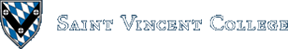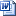Computing & Information Systems    DepartmentSearch CIS Site      Tutorials

### Spring 2020

This course emphasizes the mathematical and theoretical foundations of computer science. The primary topics are computability theory and Turing machines, complexity theory (including the classes P, NP, NP-complete, and NP hard), grammars and parsing, push-down automata, and running time analysis (especially using recurrence relations and generating functions). Important fundamental questions will be answered, such as whether all functions are computable and the existence of unsolvable problems. Also included is an introduction to proofs of program correctness and some running time analysis for algorithms to solve the traveling salesperson problem.

### Further Information

 Course Syllabus Documents, homilies, and speeches of Pope Francis Poverty, Hunger, and Social Justice in AmericaIn honor of Martin Luther King, Jr. Parser Freeware and Examples Wolfram Mathematica Documentation Center Wolfram Learning Center Wolfram MathWorld Wolfram Functions Wolfram Library Wolfram Demonstrations The Shroeder-Bernstein Theorem Education web site by Texas Instruments Includes manuals and tutorials for many TI calculators and links to math web sites. Example Prolog programsIncluding tictac.pro. Br. David's Traveling Salesperson Problem: Software and Lab webpage. AMS Overview of the TSP Neil Simonetti's TSP Page Using Mathematica to Solve a TSP RSA.com RSA Conference AlanTuring.net ACM Code of Ethics All Elementary Mathematics See the algebra section for mathematical induction, sequences, permutations and combinations, etc. The Probability Web: Teaching Resources This site has lots of links to resources on probability and statistics. The Math Forum: Discrete Mathematics National Curve Bank Textbook web siteProvides guides to writing proofs and common mistakes in discrete mathematics, links to external Web sites, extra examples, self-assessment on some key topics, and interactive demonstrations of important algorithms. Example proofsThe status of the P versus NP Problem P versus NP problem LearnSAT is a satisfiability solver for propositional logic formulas. Although the SAT problem is NP-complete, this and other SAT solvers are said to perform well. Godel's Incompleteness Theorems The probabilistic checkable proofs theorem is one of the big developments in recent years in the area of complexity theory. In recent years, the concept of continuous programs has been developed. See, for example, the article Proving Programs Continuous or Continuity and Robustness of Programs

### Homework and Announcements

 Now in Schoology

#### Instructor: Br. David Carlson

Maintained by: Br. David Carlson
Last updated: January 15, 2020
Disclaimer